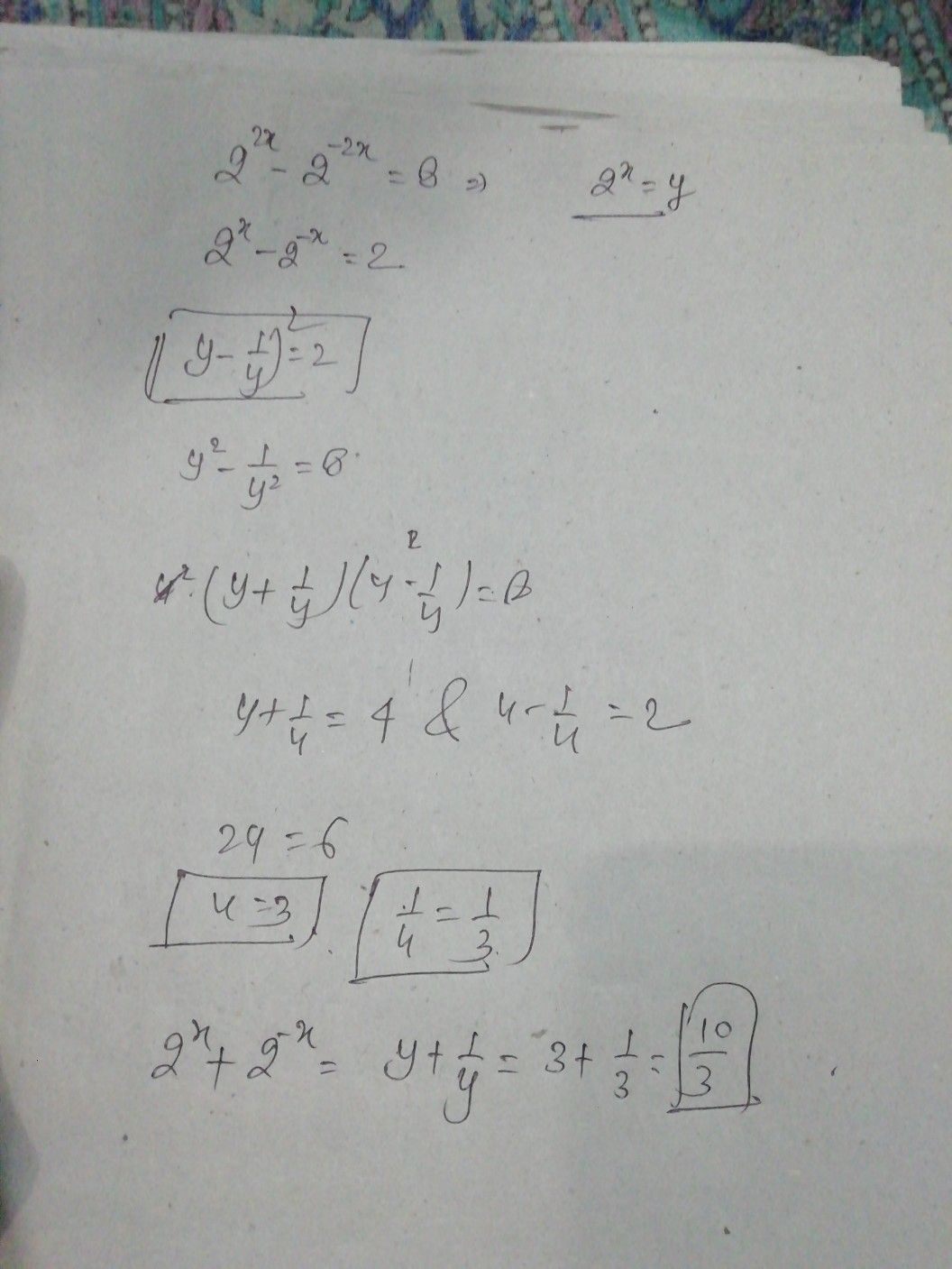Symbol
Problem$2^{2x}-2-2x=8$ $2^{x}-2-x=2$ $\left(=2^{x}+2-x=7$